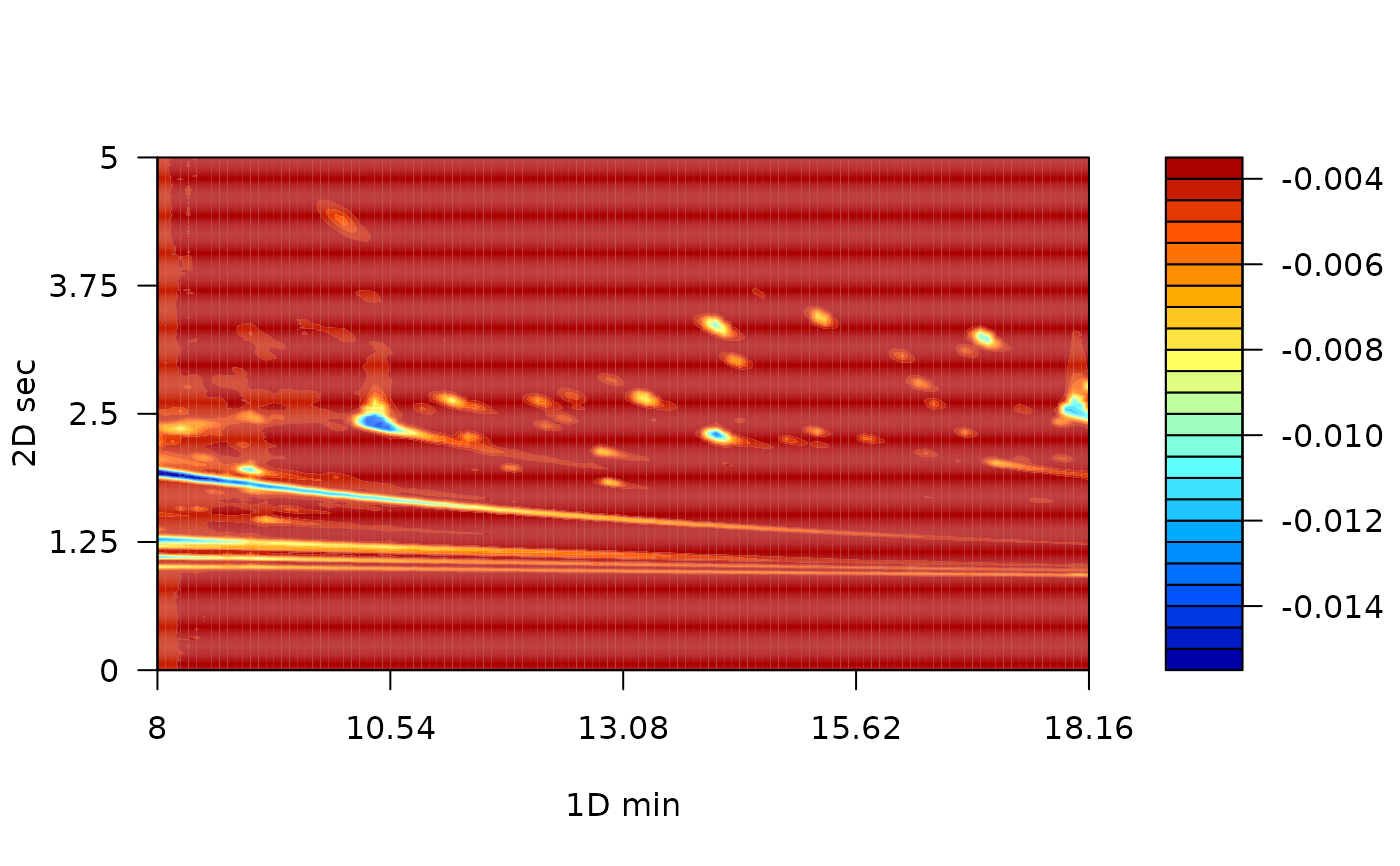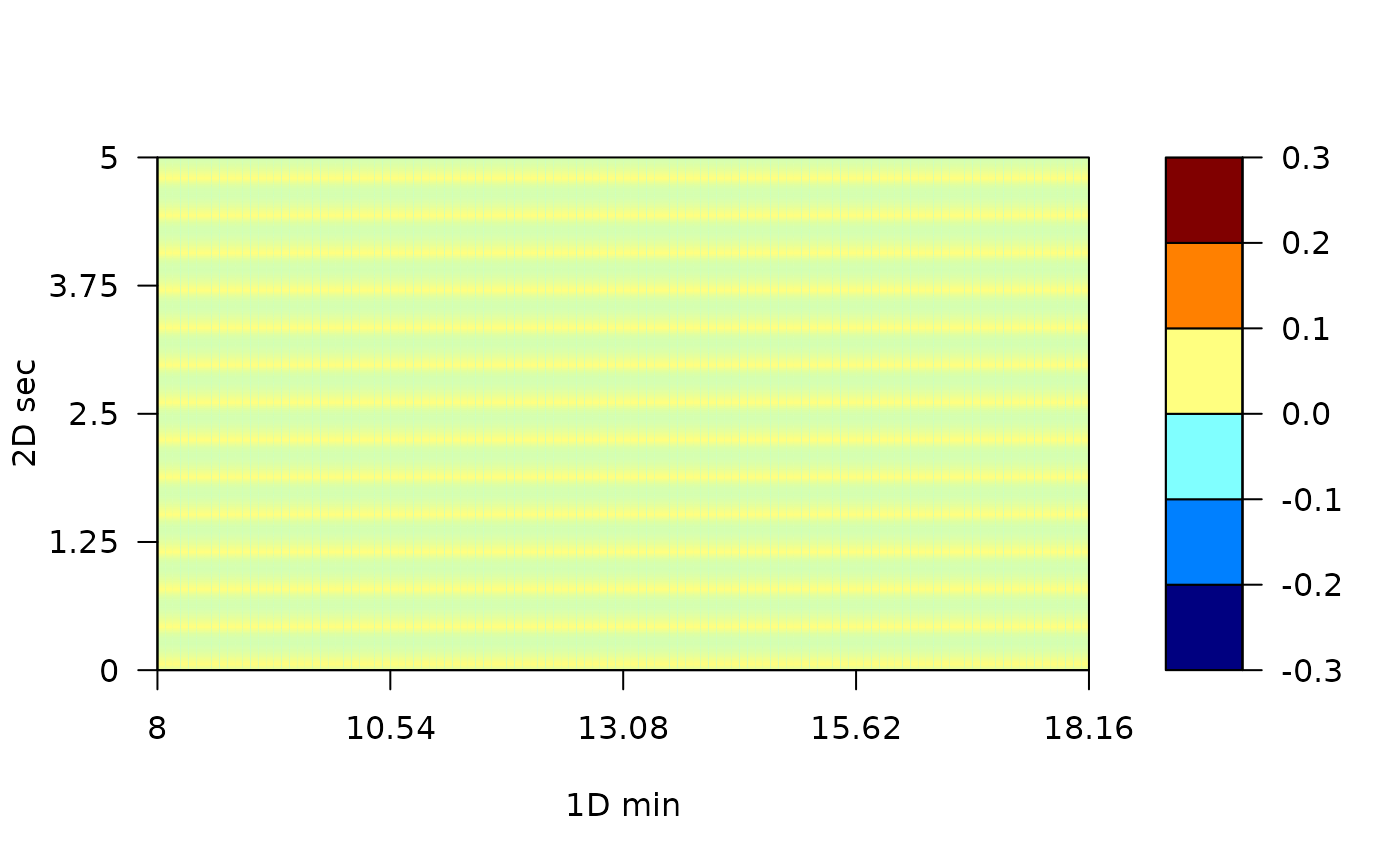plot_loading plot the loading of the a MPCA object.

## Usage

plot_loading(Object, type = "n", pc = 1, thresh, ...)

# S4 method for projected
plot_loading(Object, type = "b", pc = 1, thresh, ...)

## Arguments

Object

a MPCA object

type

pc

the principal component to plot.

thresh

...

Other parameters passes to filled.contour function.

## Details

This function takes the loadings of MPCA and eval if a certain variable was removed previous compute de MPCA and it fills the removed variables with zero. Then, the loadings are plotted considering one principle component at a time as a two-dimensional chromatogram.

## Examples


library(colorRamps)
data(MTBLS579)
# MPCA with mean-centered and scaled data
MTBLS579_mpca <- m_prcomp(MTBLS579)